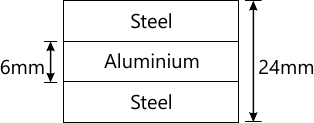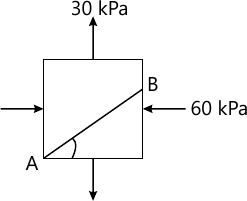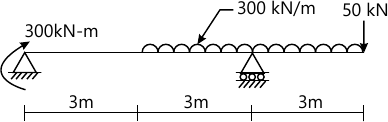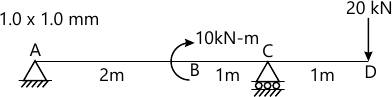MORE IN Strength of Materials
VTU Civil Engineering (Semester 3)
Strength of Materials
December 2014
Total marks: --
Total time: --
INSTRUCTIONS
(1) Assume appropriate data and state your reasons
(2) Marks are given to the right of every question
(3) Draw neat diagrams wherever necessary

1 (a) Draw a neat sketch of stress-strain curve for mild steel specimen in tension. Mark the salient points on it.
5 M
1 (b) Derive the relationship between Young's modulus, and bulk modulus of a material.
5 M
1 (c) A 1.5 m long steel bar having uniform diameter of 40 mm for a length of 1 m and in the next 0.5 m its diameter gradually reduces to 20 mm. Determine the elongation of the bar when subjected to an axial tensile load of 160 kN. Take E=200 Gpa.
10 M

2 (a) A concrete column is of square section with 250 mm size and is reinforced with 08 steel bars of 16 mm diameter. The member supports an axial load of 270 kN. Evaluate the stresses in steel and concrete assuming a modular radio as 18.
8 M
2 (b) A flat bar of aluminium alloy 24 mm wide and 6 mm thick is placed between steel bars each 24mm wide and 9 mm thick to form a composite bar (24×24)mm as shown in fig. 2(b). The three bars are fastened together at their ends when the temperature is 10°C. Find the stresses in each of the material when the temperature of the whole assembly is raised to 50°C. If at the new temperature a compressive load of 20 kN is applied to the composite bar, What are the final stresses in steel and aluminium? Take Es=2×105 N/mm2, Ea=2/37times;105 N/mm2, αs=1.2×10-5 per degree C; aa=2.3×10-5 per degree C.12 M

3 (a) Define principal stresses and principle planes.
3 M
3 (b) A rectangular block of materials is subjected to tensile stresses of 120 N/mm2 and 60 N/mm2 on mutually perpendicular planes together with a shear stress of 70 N/mm2 Find:
i) The principal stresses
ii) The principle planes
iii) The maximum shear stress.
Verify the results by constructing Mohr's circle.
12 M
3 (c) The stresses acting in a strained material is as shown in Fig. 3(c). Find the normal and tangential stress acting on a plane AB.5 M

4 (a) Derive the relationship between intensity of load, shear force and bending Moment.
5 M
4 (b) Show that the maximum bending moment in a beam subjected to udl throughout is wl2/8, with usual notations.
5 M
4 (c) For the beam shown in Fig. 4(c), draw shear force and bending moment diagram. Mark the values at salient points.10 M

5 (a) Explain the terms 'beam of uniform strength' with the help of neat sketches.
3 M
5 (b) Define:
i) Neutral axis
ii) Section modulus
iii) Flexural rigidity
iv) Modulus of rupture
6 M
5 (c) A rolled steel joist of T-section used as a simply supported beam has the following dimensions: flange- (250× 25) mm, web -15 mm thick, overall depth - 50 mm. If this beam carries a UDL of 50 kN/m on a span of 4m; calculate maximum stress produced due to bending.
11 M

6 (a) Determine the slope and deflection for a cantilever beam subjected to clockwise moment at its free end.
8 M
6 (b) Determine the deflection at B and D for the beam shown in Fig. Q6(b). Take E=210 GPa and I=1.6×107 mm4.12 M

7 (a) Derive the expression for the theory of pure torsion, with usual notations.
8 M
7 (b) A solid circular shaft has to transmit power of 1000 kW at 120 rpm. Find the dia of the shaft if the shear stress must not exceed 80 N/mm2. Maximum torque is 1.25 times the mean, what percentage in material could be obtained if the shaft is replaced by a hollow one whose internal dia is 0.6 times the external dia. The length of material and maximum shear stress being same.
12 M

8 (a) Write short notes on limitations of Euler's formula.
5 M
8 (b) Define buckling load slenderness ratio.
3 M
8 (c) Compare the crippling loads given by Euler's and Rankinn's formula for a tubular steel column 2.5 m long having outer and inner dia as 40mm and 30 mm respectively loaded through pin jointed ends. Take yield stress=320 N/mm2, a=1/7500 and E=210 GPa. For what length of the column this cross section the Euler's formula cease to apply?
12 M

More question papers from Strength of Materials